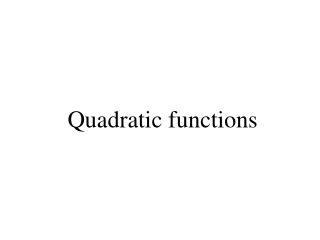DownloadDownload PresentationTélécharger la présentation- - - - - - - - - - - - - - - - - - - - - - - - - - - E N D - - - - - - - - - - - - - - - - - - - - - - - - - - -
##### Presentation Transcript

2. Quadratic functions: example Group excursion • Minimum 20 participants • Price of the guide: 122 EUR • For 20 participants: 80 EUR per person • For every supplementary participant: for everybody (also the first 20) a price reduction of 2 EUR per supplementary participant Revenue of the travel agency when there are 6 supplementary participants? total revenue = 122 + (20 + 6)  (80  2  6) = 1890

3. Quadratic functions: example Group excursion • Minimum 20 participants • Price of the guide: 122 EUR • For 20 participants: 80 EUR per person • For every supplementary participant: for everybody (also the first 20) a price reduction of 2 EUR per supplementary participant Revenue y of the travel agency when there are x supplementary participants? y = 122 + (20 + x)(80  2x) =  2x²+ 40x + 1722 QUADRATIC FUNCTION!

4. Quadratic functions: 3 representations Equation: Graph: PARABOLA Table:

5. Quadratic functions: equation A function f (“rule”) with an equation of the form f(x) = y=ax² + bx + c where a  0 is called a quadratic function

6. Quadratic equations Revenue equal to 1872?  2x²+ 40x + 1722 = 1872 • 2x²+ 40x + 1722  1872 = 0 • 2x²+ 40x 150 = 0 We have to solve the equation  2x²+ 40x 150 = 0. Finding SOLUTIONS of an equation of the form ax² + bx + c = 0 QUADRATIC EQUATION

7. Quadratic functions: definitions • Function f (“MACHINE”!) with an equation of the form f(x) = ax² + bx + c where a  0. Or: function having an explicit equation of the formy = ax² + bx + c where a  0. • Discriminant: d = b²  4ac

8. Quadratic equations • Equations that can be written in the form ax² + bx + c = 0 where a0. • Solutions: if discriminant d > 0: two solutions if discriminant d = 0: one solution if discriminant d < 0: no solutions Discriminant: d = b²  4ac  2x²+ 40x 150 = 0 Group excursion:

9. Quadratic functions: exercises • exercise 1 (a), (c) and (e) • supplementary exercises: rest of exercise 1

10. Quadratic function: graph Quadratic functions: graph is a PARABOLA Graphical interpretation of y=ax²+bx+c=0 ? Zero’s, solutions of this quadratic equations correspond to intersections with the horizontal x-axis Sign of the discriminant determines the number of intersections with the horizontal axis

11. Quadratic function: graph sign of the discriminant determines the number of intersections with the horizontal axis sign of the coefficient of x 2 determines the orientation of the opening

12. Quadratic function: maximum Maximum revenue? In this case you can find it e.g. using the table: So: 10 supplementary participants (30 participants in total) This can also be determined algebraically, based on a general study of quadratic functions!

13. Quadratic function: maximum x-coordinate of the vertex of the parabola: the vertex determines the minimum/maximum function value Group excursion:

14. Quadratic functions: exercises • Exercise 2 (f1 and f5) • Exercise 3, 7, 5 supplementary exercises: • rest of exercise 2 • exercise 4, 6, 8 and 9 Figure

15. Quadratic inequalities Solve the inequality i.e. Find all x for which standard form graph of LHS conclusion: x-2 or x7 interval notation: ]-,-2][7,[

16. Quadratic inequalities inequalities that can be reduced to the form ... and determine the common points with the x-axis by solving the EQUATION

17. Quadratic functions: exercises • Exercise 10 (a) • Exercises 11 (a), (c) Supplementary exercises: • Exercises 10 (b), (c), (d) • Exercises 11 (b), (d)

18. Quadratic functions: Summary • 3 representations : table, equation, graph • Quadratic equations , zero’s, discriminant d • Graph: Parabola interpretation of d, a Maximum, vertex • Quadratic inequalities

19. STUDYING MATH is DOING A LOT OF EXERCISES YOURSELF, MAKING MISTAKES AND DOING THE EXERCISES AGAIN CORECTLY

20. Exercise 7 Back